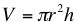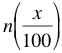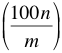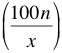# Math Help: Useful Formulas to Learn

09/21/2015

## Math Formulas You Should Know by Heart

Math builds on the knowledge you have gained before, so there are certain formulas you should memorize to help you advance. Here is a list of a few of them.

### Triangle Formulas

Area=1/2(b*h) Think of a triangle as half of a rectangle, so you need to take half of the base time height. The base of the triangle is the length of a side. If you have a right triangle, the height is the length of the side with the 90 degree angle. If non-right triangle, drop a line from the apex of the triangle to the base. Isosceles triangles have two equal sides and two equal angles. If one of the angles is 90 degrees, the other two will be 45. The lengths of a right isosceles triangle are always x, x, x√2. The find the hypotenuse, simply multiply √2 by one of the sides. The side lengths of a right triangle with angles 30-60-90 can be found using the formula x, x√3, and 2x. The smallest side, x, is opposite the smallest angle, 30. The longest side, 2x, is opposite the largest angle, 90.

### Cylinder VolumeThe volume of a cylinder is the area of the circle that makes up its end times the height of the cylinder.

### Statistical Combinations

To find the total possible different combinations of different elements, simply multiply them all together. For example, if there are 4 appetizers, 5 entrees, and 3 desserts on a menu, there are 4*5*3=60 different possible combinations.

### Percentages

To find x percent of a number (n):To find what percent a number (n) is of another number (m):And finally, n is x percentage of what number:## For Tutoring in Santa Clara, CA

The Tutoring Center in Santa Clara offers one-to-one tutoring help in math, reading, writing, and more. Our webpage will inform you of our individualized programs, but you should call 408 816 7825 to schedule a free diagnostic assessment.

### FREE CONSULTATION

Schedule your Free Diagnostic Assessment Today!